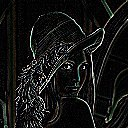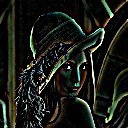## GAUSSIAN

 Generates high pass or low pass filtered images based upon a gaussian shaped convolution.

file is missing or empty

 USAGE: gaussian [-w width] [-k kind] [-m mix] infile outfile USAGE: gaussian [-h or -help] -w ...... width .......... width for Gaussian blur filter; ........................... See sigma in -blur; float > 0 ........................... default=1 -k ...... kind ............ kind=high or low (pass filter) or edge; ........................... default=high -m ...... mix ............. mixing percent with original image; ........................... mix=integer 0 to 100; default=20 -h ........................ get help information -help ..................... get help information PURPOSE: To generate high pass or low pass filtered images based upon a Gaussian shaped convolution. DESCRIPTION: GAUSSIAN generates an output image which is a user defined mix or blend of the original image and a Gaussian blurred version of the image. The basic blended low pass filtering formula is F = (1-m)*I + m*L, where I is the original image, L is the Gaussian low pass filtered image and m = mix/100. When m=0, we get only the original image and when m=1, we get only the low pass Gaussian filtered image. For intermediate value of m, we get a blend of the image and the Gaussian low pass filtered image. For high pass filtering, we form the high pass filter by subtracting the low pass filter from the original Thus in the formula above L is replaced by H=(I-L), and as the high pass image is primarily black we simply add the percentage of the high pass image to the original image so that F = I + m*H, which also can be expressed as F = (1+m)*I - m*L. For the edge image, we simply output the high pass filtered image so tht F=H. ARGUMENTS: -w width ... WIDTH is the (half) width for the Gaussian blurring (low pass) convolution filter. This corresponds to sigma for the -blur IM function with radius set to 0 so that it is determined automatically. Width is a positive floating point value. Default=1. See the function -blur in the IM documentation. -k kind ... KIND is the kind of filter, either a high pass, low pass filter or edge image are allowed. Thus kind=high, low or edge. The default=high. A low pass filter will cause blurring. A high pass filtered image will produce either sharpening and the edge option will extract edges. See below. -m mix ... MIX is the percentage mixing factor to use to blend the filtered result with the original image. For kind=low, a value of mix=0, results in the original image and a value of mix=100 results in a pure low pass filtered image. For low pass filtering, a larger value for mix will produce more blurring. For kind=high, the mix percentage of the high pass filtered image will be added to the original image. A larger value for mix will sharpen or highlight the edges more. Fr kind=edge, the mix value is not relevant or is ignored. The default is mix=20. CAVEAT: No guarantee that this script will work on all platforms, nor that trapping of inconsistent parameters is complete and foolproof. Use At Your Own Risk.

### EXAMPLES

 Low Pass Filtering Original Image Arguments: -k low -m 50 [-w 1] Arguments: -k low -m 100 [-w 1] Arguments: -k low -m 100 -w 2High Pass Filtering Original Image Arguments: -k high -m 10 [-w 1] Arguments: -k high -m 20 [-w 1] Arguments: -k high -m 30 [-w 1]Edge Extraction Original Image Arguments: -k edge -w 0.5 Arguments: -k edge -w 1 (default) Arguments: -k edge -w 2What the script does is as follows: Applies a Gaussian blurring filter to the image to form the low pass filtered image Subtracts the low pass filtered image from the origial image to form the high pass filtered image Blends the original image with either the low pass filtered image or the high pass filtered image to blur or sharpen, respectively This is equivalent to the following IM commands for the case of image sharpening: convert \$infile \( \$infile -blur 0x\$width \) -compose minus +swap \ -composite -normalize \$tmp0 composite -blend \$mix%x100% \$tmp0 \$infile -matte \$outfile